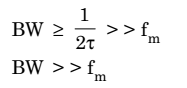# Transmission Bandwidth in Pulse Amplitude Modulation (PAM)

## Transmission Bandwidth in PAM

In a pulse amplitude modulated (PAM) signal the pulse duration ‘τ’ is considered to be very small in comparison to time period (i.e., sampling period) Ts between any two samples i.e.,

Now, if the maximum frequency in the modulating signal x(t) is fm, then according to sampling theorem, the sampling frequency fs must be equal to or higher than the Nyquist rate, i.e.,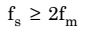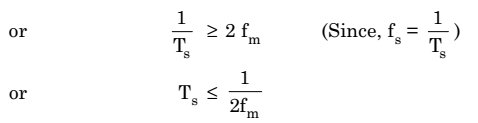But according to equation (1), we have,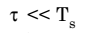Therefore,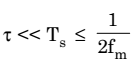Now, if the ‘ON’ and ‘OFF’ time of the pulse amplitude modulated (PAM) pulse is same then maximum frequency of the PAM pulse will be equal to,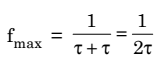Therefore, the bandwidth required for the transmission of a PAM signal would be equal to the maximum frequency fmax given by the above equation.

Thus, we have Transmission bandwidth